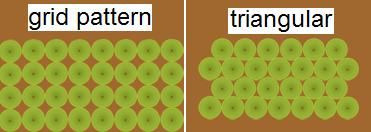Welcome to our perennial plant nursery!
Imagine More Perennial Plants Than You Can Imagine, Delivered To You.

Free Shipping to most states

# How Many Plants Do You Need?

 # of Plants On Center Spacing of Plants 8" 10" 12" 15" 18" 24" Coverage Area in Square Feet 25 57 36 25 16 11 6 50 114 72 50 32 22 12 75 171 108 75 48 33 18 100 228 144 100 64 44 24 150 342 216 150 96 66 36 200 456 288 200 128 88 48 Spacing Multiplier 2.28 1.44 1.0 0.64 0.44 0.25

For other size areas, use the following formula to calculate how many plants you will need:

`(Area in sq. feet) x (Spacing Multiplier) = Number of plants needed `
`(Area is 2 feet by 25 feet = 50 sq. feet) x (Spacing 12 inches apart Spacing Multiplier is 1.0 ) = 50 is the number of plants needed `

Finding Unknown Spacing Multiplier

For other spacing requirements, use the following formula to figure your multiplier:

Multiplier = 144/X2 where X = the spacing distance in inches you need

Example: If you need a spacing of 13.5 inches, your multiplier would be:

 144 = 144 = 0.79 `13.52` 182.25 multipleThe above chart and formula are based on planting in a grid pattern. If you are planting in a staggered or equilateral triangular pattern (which gives a more massed effect) the rows will be a little closer together and more plants will be needed.
An additional 15% will be required and the row spacing would be reduce by a factor of 0.866 (the sine of 60 degrees) for the equilateral triangular layout.
For example - based on 100 square feet and a spacing of 12 inches, 100 plants are needed. In a trianglar pattern 15 more plants would be needed (100 x 15% = 15). Instead of 12 inch rows, rows would be 10.4 inches apart (12 x .866 = 10.392).